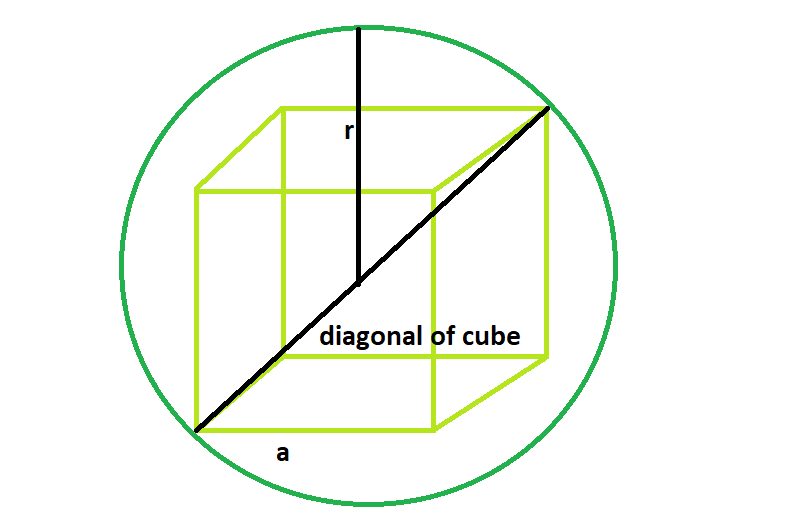# Largest cube that can be inscribed within the sphere

Given here is a sphere of radius r, the task is to find the side of the largest cube that can fit inside in it.

Examples:

```Input: r = 8
Output: 9.2376

Input: r = 5
Output: 5.7735
```

## Recommended: Please try your approach on {IDE} first, before moving on to the solution.Approach:

Side of the cube = a
Radius of the sphere = r
From the diagonal, it is clear that, diagonal of the cube = diameter of the sphere,
a√3 = 2r or, a = 2r/√3

Below is the implementation:

## C++

 `// C++ Program to find the biggest cube ` `// inscribed within a sphere ` `#include ` `using` `namespace` `std; ` ` `  `// Function to find the side of the cube ` `float` `largestCube(``float` `r) ` `{ ` ` `  `    ``// radius cannot be negative ` `    ``if` `(r < 0) ` `        ``return` `-1; ` ` `  `    ``// side of the cube ` `    ``float` `a = (2 * r) / ``sqrt``(3); ` `    ``return` `a; ` `} ` ` `  `// Driver code ` `int` `main() ` `{ ` `    ``float` `r = 5; ` `    ``cout << largestCube(r) << endl; ` ` `  `    ``return` `0; ` `} `

## Java

 `// Java Program to find the biggest cube  ` `// inscribed within a sphere  ` `import` `java.util.*; ` `class` `Solution{ ` `// Function to find the side of the cube  ` `static` `float` `largestCube(``float` `r)  ` `{  ` `   `  `    ``// radius cannot be negative  ` `    ``if` `(r < ``0``)  ` `        ``return` `-``1``;  ` `   `  `    ``// side of the cube  ` `    ``float` `a = (``2` `* r) / (``float``)Math.sqrt(``3``);  ` `    ``return` `a;  ` `}  ` `   `  `// Driver code  ` `public` `static` `void` `main(String args[]) ` `{  ` `    ``float` `r = ``5``;  ` `    ``System.out.println( largestCube(r));  ` `   `  `}  ` ` `  `} ` `//contributed by Arnab Kundu `

## Python3

 `# Python 3 Program to find the biggest  ` `# cube inscribed within a sphere ` `from` `math ``import` `sqrt ` ` `  `# Function to find the side of the cube ` `def` `largestCube(r): ` `     `  `    ``# radius cannot be negative ` `    ``if` `(r < ``0``): ` `        ``return` `-``1` ` `  `    ``# side of the cube ` `    ``a ``=` `(``2` `*` `r) ``/` `sqrt(``3``) ` `    ``return` `a ` ` `  `# Driver code ` `if` `__name__ ``=``=` `'__main__'``: ` `    ``r ``=` `5` `    ``print``(``"{0:.6}"``.``format``(largestCube(r))) ` ` `  `# This code is contributed ` `# by SURENDRA_GANGWAR `

## C#

 `// C# Program to find the biggest cube  ` `// inscribed within a sphere  ` `using` `System; ` `class` `Solution{ ` `// Function to find the side of the cube  ` `static` `float` `largestCube(``float` `r)  ` `{  ` ` `  `    ``// radius cannot be negative  ` `    ``if` `(r < 0)  ` `        ``return` `-1;  ` ` `  `    ``// side of the cube  ` `    ``float` `a = (2 * r) / (``float``)Math.Sqrt(3);  ` `    ``return` `a;  ` `}  ` ` `  `// Driver code  ` `static` `void` `Main() ` `{  ` `    ``float` `r = 5;  ` `    ``Console.WriteLine( largestCube(r));  ` ` `  `}  ` ` `  `} ` `//This code is contributed by mits `

## PHP

 ` `

Output:

```5.7735
```

Attention reader! Don’t stop learning now. Get hold of all the important DSA concepts with the DSA Self Paced Course at a student-friendly price and become industry ready.

My Personal Notes arrow_drop_upBudding Web DeveloperKeen learnerAverage CoderDancer&Social Activist

If you like GeeksforGeeks and would like to contribute, you can also write an article using contribute.geeksforgeeks.org or mail your article to contribute@geeksforgeeks.org. See your article appearing on the GeeksforGeeks main page and help other Geeks.

Please Improve this article if you find anything incorrect by clicking on the "Improve Article" button below.

Article Tags :
Practice Tags :

Be the First to upvote.

Please write to us at contribute@geeksforgeeks.org to report any issue with the above content.